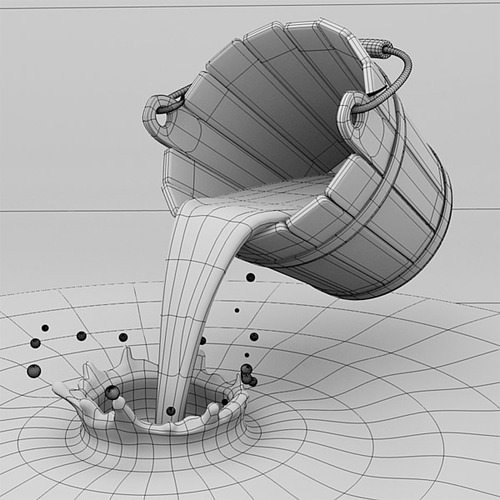# Making bucket empty with 50% take out rate puzzle

1,181.1K Views

A bucket is full of water.  50% of the water is taken from it every hour.
How many hours will it take until  it is completely empty?

Hint:- Answer is not infinite 🙂EXPLANATION-
Surprising as it may seem, the answer follows from the properties of matter and Avogadro’s number.

All the answers provided here so far assume that the bucket of water is infinitely divisible. But the fact is there comes a stage where you are left with just one molecule in the bucket. You cannot divide a single molecule any further without losing the property of water. It is a fundamental principle of chemistry. You stop when the bucket has just one molecule or you remove that one molecule also after one hour.

With that background, let us look at the calculations.
One mole of water weighs 18.015 grams.
One mole of water (any substance for that matter) contains 6.022 x 10^23 molecules (Avogadro’s number).
To keep the calculations simple, let us assume the bucket holds 36 litres or 36 kilograms  of water (that is a reasonable size also).
36 kgs of water is equal to 2000 moles (approx).
2000 moles of water contain 2000 x 6.022 x 10^23  = 1.2044e+27 molecules.
hours  Molecules
0        1.2044e+27
1         6.022E+26
2         3.011E+26
3         1.505E+26
.
.
.
87       7.783E+00
88       3.892E+00
89       1.946E+00
90       9.729E-01 (Note the sign has flipped from + to -)

You can also start with one molecule at zero hours, double the quantity every hour and see how long it takes to reach 1.2044e+27 molecules.

If you double the size of the bucket, the time goes up by one more hour.

Here is the python script that did the actual calculation:

molecules = 2000 * 6.022 * 10 ** 23
hours = 0
while molecules > 1:
molecules = molecules / 2
hours = hours + 1
print hours, ‘%.3E’ % molecules

SourceQuora